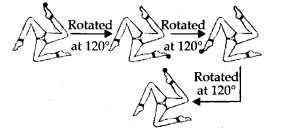# MP Board Class 7th Maths Solutions Chapter 14 Symmetry Ex 14.2

## MP Board Class 7th Maths Solutions Chapter 14 Symmetry Ex 14.2

Question 1.
Which of the following figures have rotational symmetry of order more than 1 :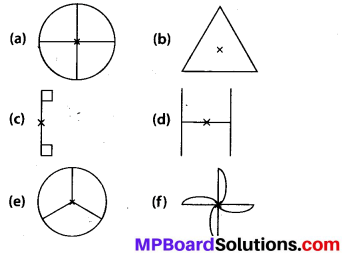Solution:
(a) The given figure has its rotational symmetry of order 4.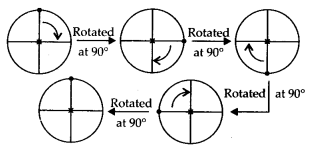(b) The given figure has its rotational symmetry of order 3.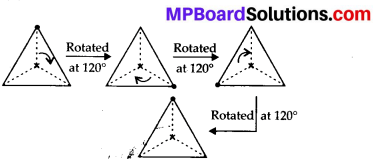(c) The given figure has its rotational symmetry of order 1.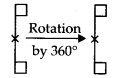(d) The given figure has its rotational symmetry of order 2.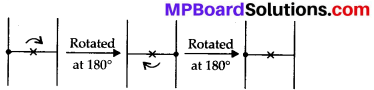(e) The given figure has its rotational symmetry of order 3.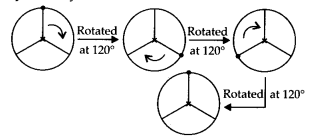(f) The given figure has its rotational symmetry of order 4.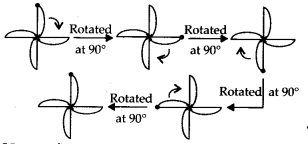Hence, figures (a), (b), (d), (e) and (f) have rotational symmetry of order more than 1.Question 2.
Give the order of rotational symmetry for each figure: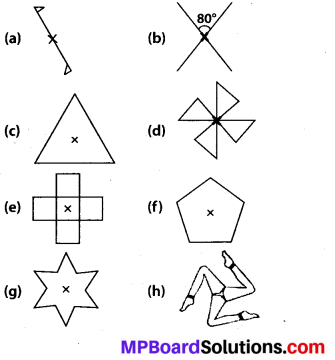Solution:
(a) The given figure has rotational symmetry of order 1.(b) The given figure has rotational symmetry of order 2.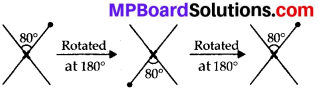(c) The given figure has rotational symmetry of order 3.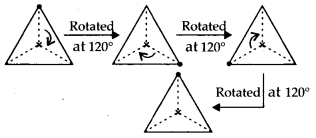(d) The given figure has rotational symmetry of order 4.(e) The given figure has rotational symmetry of order 4.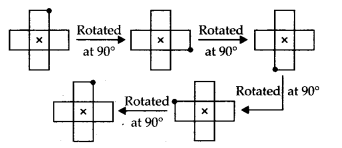(f) The given figure has rotational symmetry of order 5.(g) The given figure has rotational symmetry of order 6.(h) The given figure has rotational symmetry of order 3.